# Represent Nonlinear Dynamics Using MATLAB File for Grey-Box Estimation

This example shows how to construct, estimate and analyze nonlinear grey-box models.

Nonlinear grey-box (`idnlgrey`) models are suitable for estimating parameters of systems that are described by nonlinear state-space structures in continuous or discrete time. You can use both `idgrey` (linear grey-box model) and `idnlgrey` objects to model linear systems. However, you can only use `idnlgrey` to represent nonlinear dynamics. To learn about linear grey-box modeling using `idgrey`, see Building Structured and User-Defined Models Using System Identification Toolbox.

In this example, you model the dynamics of a linear DC motor using the `idnlgrey` object.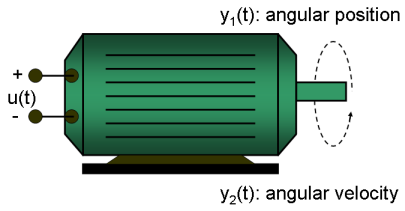Figure 1: Schematic diagram of a DC-motor.

If you ignore the disturbances and choose `y(1)` as the angular position [rad] and `y(2)` as the angular velocity [rad/s] of the motor, you can set up a linear state-space structure of the following form (see Ljung, L. System Identification: Theory for the User, Upper Saddle River, NJ, Prentice-Hall PTR, 1999, 2nd ed., p. 95-97 for the derivation):

``` d | 0 1 | | 0 | -- x(t) = | | x(t) + | | u(t) dt | 0 -1/tau | | k/tau |```
``` | 1 0 | y(t) = | | x(t) | 0 1 |```

`tau` is the time-constant of the motor in [s] and `k` is the static gain from the input to the angular velocity in [rad/(V*s)] . See Ljung (1999) for how `tau` and `k` relate to the physical parameters of the motor.

1. Load the DC motor data.

```load('dcmotordata'); ```

2. Represent the estimation data as an `iddata` object.

```z = iddata(y, u, 0.1, 'Name', 'DC-motor'); ```

3. Specify input and output signal names, start time and time units.

```z.InputName = 'Voltage'; z.InputUnit = 'V'; z.OutputName = {'Angular position', 'Angular velocity'}; z.OutputUnit = {'rad', 'rad/s'}; z.Tstart = 0; z.TimeUnit = 's'; ```

4. Plot the data.

The data is shown in two plot windows.

```figure('Name', [z.Name ': Voltage input -> Angular position output']); plot(z(:, 1, 1)); % Plot first input-output pair (Voltage -> Angular position). figure('Name', [z.Name ': Voltage input -> Angular velocity output']); plot(z(:, 2, 1)); % Plot second input-output pair (Voltage -> Angular velocity). ```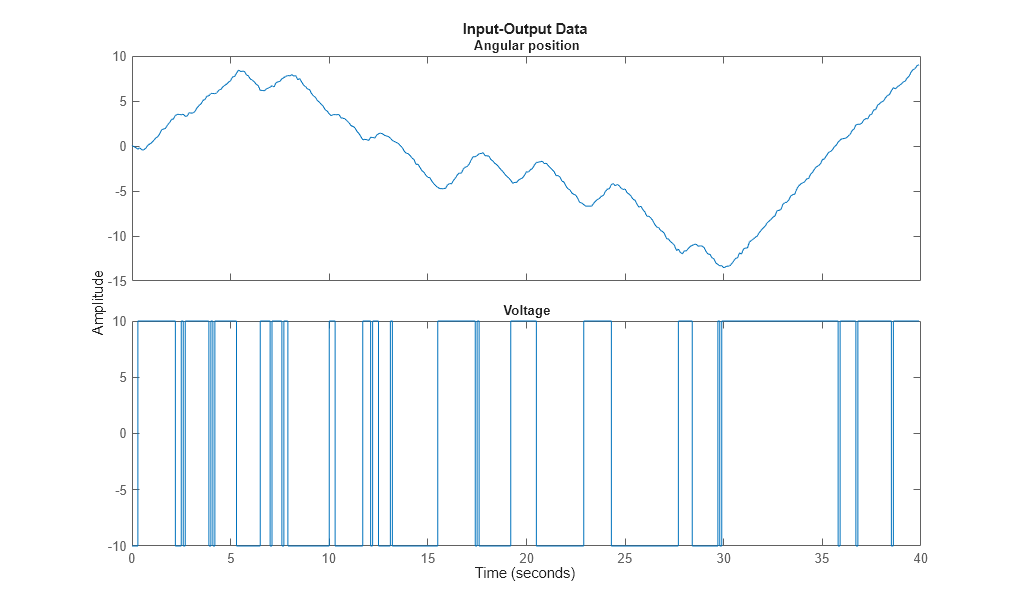Figure 2: Input-output data from a DC-motor.

### Linear Modeling of the DC-Motor

1. Represent the DC motor structure in a function.

In this example, you use a MATLAB® file, but you can also use C MEX-files (to gain computational speed), P-files or function handles. For more information, see Creating IDNLGREY Model Files.

The DC-motor function is called `dcmotor_m.m` and is shown below.

` function [dx, y] = dcmotor_m(t, x, u, tau, k, varargin)`
``` % Output equations. y = [x(1); ... % Angular position. x(2) ... % Angular velocity. ];```
``` % State equations. dx = [x(2); ... % Angular velocity. -(1/tau)*x(2)+(k/tau)*u(1) ... % Angular acceleration. ];```

The file must always be structured to return the following:

Output arguments:

• `dx` is the vector of state derivatives in continuous-time case, and state update values in the discrete-time case.

• `y` is the output equation

Input arguments:

• The first three input arguments must be: `t` (time), `x` (state vector, [] for static systems), `u` (input vector, [] for time-series).

• Ordered list of parameters follow. The parameters can be scalars, column vectors, or 2-dimensional matrices.

• `varargin` for the auxiliary input arguments

2. Represent the DC motor dynamics using an `idnlgrey` object.

The model describes how the inputs generate the outputs using the state equation(s).

```FileName = 'dcmotor_m'; % File describing the model structure. Order = [2 1 2]; % Model orders [ny nu nx]. Parameters = [1; 0.28]; % Initial parameters. Np = 2. InitialStates = [0; 0]; % Initial initial states. Ts = 0; % Time-continuous system. nlgr = idnlgrey(FileName, Order, Parameters, InitialStates, Ts, ... 'Name', 'DC-motor'); ```

In practice, there are disturbances that affect the outputs. An `idnlgrey` model does not explicitly model the disturbances, but assumes that these are just added to the output(s). Thus, `idnlgrey` models are equivalent to Output-Error (OE) models. Without a noise model, past outputs do not influence prediction of future outputs, which means that predicted output for any prediction horizon k coincide with simulated outputs.

3. Specify input and output names, and units.

```set(nlgr, 'InputName', 'Voltage', 'InputUnit', 'V', ... 'OutputName', {'Angular position', 'Angular velocity'}, ... 'OutputUnit', {'rad', 'rad/s'}, ... 'TimeUnit', 's'); ```

4. Specify names and units of the initial states and parameters.

```nlgr = setinit(nlgr, 'Name', {'Angular position' 'Angular velocity'}); nlgr = setinit(nlgr, 'Unit', {'rad' 'rad/s'}); nlgr = setpar(nlgr, 'Name', {'Time-constant' 'Static gain'}); nlgr = setpar(nlgr, 'Unit', {'s' 'rad/(V*s)'}); ```

You can also use `setinit` and `setpar` to assign values, minima, maxima, and estimation status for all initial states or parameters simultaneously.

5. View the initial model.

a. Get basic information about the model.

The DC-motor has 2 (initial) states and 2 model parameters.

```size(nlgr) ```
```Nonlinear grey-box model with 2 outputs, 1 inputs, 2 states and 2 parameters (2 free). ```

b. View the initial states and parameters.

Both the initial states and parameters are structure arrays. The fields specify the properties of an individual initial state or parameter. Type `help idnlgrey.InitialStates` and `help idnlgrey.Parameters` for more information.

```nlgr.InitialStates(1) nlgr.Parameters(2) ```
```ans = struct with fields: Name: 'Angular position' Unit: 'rad' Value: 0 Minimum: -Inf Maximum: Inf Fixed: 1 ans = struct with fields: Name: 'Static gain' Unit: 'rad/(V*s)' Value: 0.2800 Minimum: -Inf Maximum: Inf Fixed: 0 ```

c. Retrieve information for all initial states or model parameters in one call.

For example, obtain information on initial states that are fixed (not estimated) and the minima of all model parameters.

```getinit(nlgr, 'Fixed') getpar(nlgr, 'Min') ```
```ans = 2x1 cell array {} {} ans = 2x1 cell array {[-Inf]} {[-Inf]} ```

d. Obtain basic information about the object:

```nlgr ```
```nlgr = Continuous-time nonlinear grey-box model defined by 'dcmotor_m' (MATLAB file): dx/dt = F(t, u(t), x(t), p1, p2) y(t) = H(t, u(t), x(t), p1, p2) + e(t) with 1 input(s), 2 state(s), 2 output(s), and 2 free parameter(s) (out of 2). Name: DC-motor Status: Created by direct construction or transformation. Not estimated. ```

Use `get` to obtain more information about the model properties. The `idnlgrey` object shares many properties of parametric linear model objects.

```get(nlgr) ```
``` FileName: 'dcmotor_m' Order: [1x1 struct] Parameters: [2x1 struct] InitialStates: [2x1 struct] FileArgument: {} SimulationOptions: [1x1 struct] TimeVariable: 't' NoiseVariance: [2x2 double] InputName: {'Voltage'} InputUnit: {'V'} InputGroup: [1x1 struct] OutputName: {2x1 cell} OutputUnit: {2x1 cell} OutputGroup: [1x1 struct] Notes: [0x1 string] UserData: [] Name: 'DC-motor' Ts: 0 TimeUnit: 'seconds' Report: [1x1 idresults.nlgreyest] ```

### Performance Evaluation of the Initial DC-Motor Model

Before estimating the parameters `tau` and `k`, simulate the output of the system with the parameter guesses using the default differential equation solver (a Runge-Kutta 45 solver with adaptive step length adjustment). The simulation options are specified using the "SimulationOptions" model property.

1. Set the absolute and relative error tolerances to small values (`1e-6` and `1e-5`, respectively).

```nlgr.SimulationOptions.AbsTol = 1e-6; nlgr.SimulationOptions.RelTol = 1e-5; ```

2. Compare the simulated output with the measured data.

`compare` displays both measured and simulated outputs of one or more models, whereas `predict`, called with the same input arguments, displays the simulated outputs.

The simulated and measured outputs are shown in a plot window.

```compare(z, nlgr); ```Figure 3: Comparison between measured outputs and the simulated outputs of the initial DC-motor model.

### Parameter Estimation

Estimate the parameters and initial states using `nlgreyest`, which is a prediction error minimization method for nonlinear grey box models. The estimation options, such as the choice of estimation progress display, are specified using the "nlgreyestOptions" option set.

```nlgr = setinit(nlgr, 'Fixed', {false false}); % Estimate the initial states. opt = nlgreyestOptions('Display', 'on'); nlgr = nlgreyest(z, nlgr, opt); ```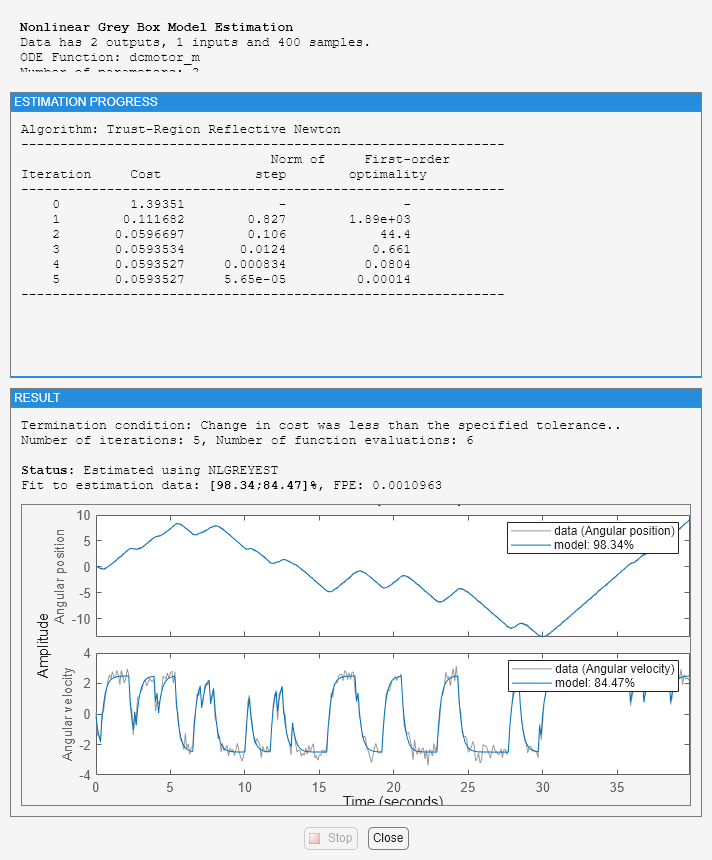### Performance Evaluation of the Estimated DC-Motor Model

1. Review the information about the estimation process.

This information is stored in the `Report` property of the `idnlgrey` object. The property also contains information about how the model was estimated, such as solver and search method, data set, and why the estimation was terminated.

```nlgr.Report fprintf('\n\nThe search termination condition:\n') nlgr.Report.Termination ```
```ans = Status: 'Estimated using NLGREYEST' Method: 'Solver: ode45; Search: lsqnonlin' Fit: [1x1 struct] Parameters: [1x1 struct] OptionsUsed: [1x1 idoptions.nlgreyest] RandState: [] DataUsed: [1x1 struct] Termination: [1x1 struct] The search termination condition: ans = struct with fields: WhyStop: 'Change in cost was less than the specified tolerance.' Iterations: 5 FirstOrderOptimality: 1.4014e-04 FcnCount: 6 Algorithm: 'trust-region-reflective' ```

2. Evaluate the model quality by comparing simulated and measured outputs.

The fits are 98% and 84%, which indicate that the estimated model captures the dynamics of the DC motor well.

```compare(z, nlgr); ```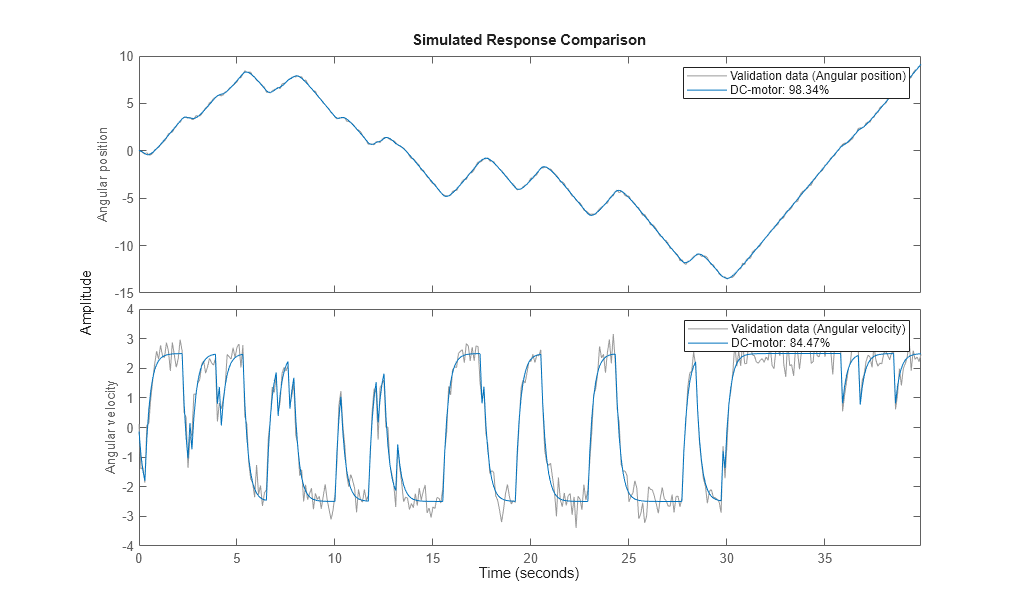Figure 4: Comparison between measured outputs and the simulated outputs of the estimated IDNLGREY DC-motor model.

3. Compare the performance of the `idnlgrey` model with a second-order ARX model.

```na = [2 2; 2 2]; nb = [2; 2]; nk = [1; 1]; dcarx = arx(z, [na nb nk]); compare(z, nlgr, dcarx); ```Figure 5: Comparison between measured outputs and the simulated outputs of the estimated IDNLGREY and ARX DC-motor models.

4. Check the prediction errors.

The prediction errors obtained are small and are centered around zero (non-biased).

```pe(z, nlgr); ```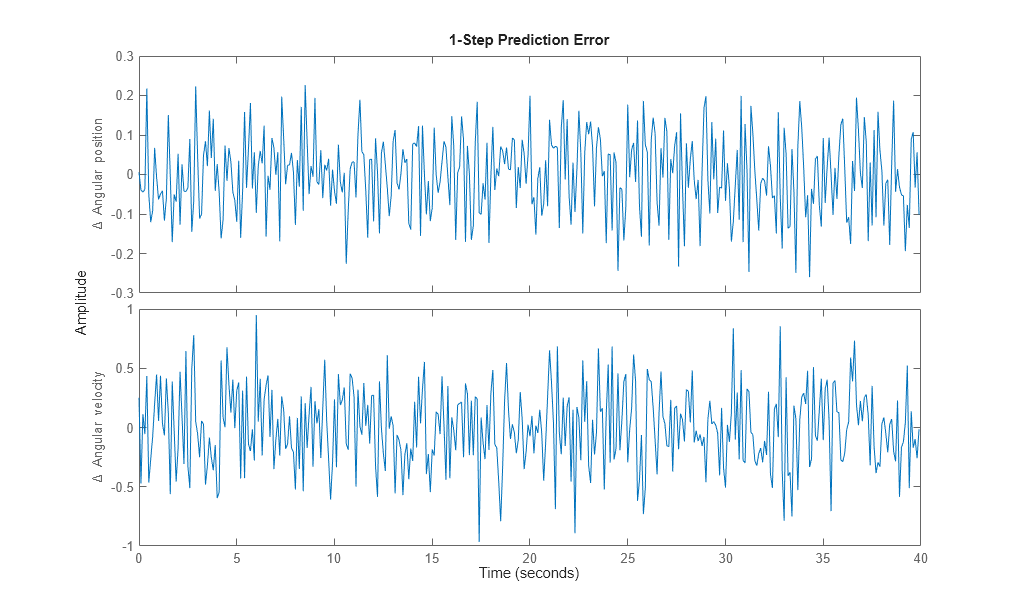Figure 6: Prediction errors obtained with the estimated IDNLGREY DC-motor model.

5. Check the residuals ("leftovers").

Residuals indicate what is left unexplained by the model and are small for good model quality. Use the `resid` command to view the correlations among the residuals. The first column of plots shows the autocorrelations of the residuals for the two outputs. The second column shows the cross-correlation of these residuals with the input "Voltage". The correlations are within acceptable bounds (blue region).

```figure('Name',[nlgr.Name ': residuals of estimated model']); resid(z,nlgr); ```Figure 7: Residuals obtained with the estimated IDNLGREY DC-motor model.

6. Plot the step response.

A unit input step results in an angular position showing a ramp-type behavior and to an angular velocity that stabilizes at a constant level.

```figure('Name', [nlgr.Name ': step response of estimated model']); step(nlgr); ```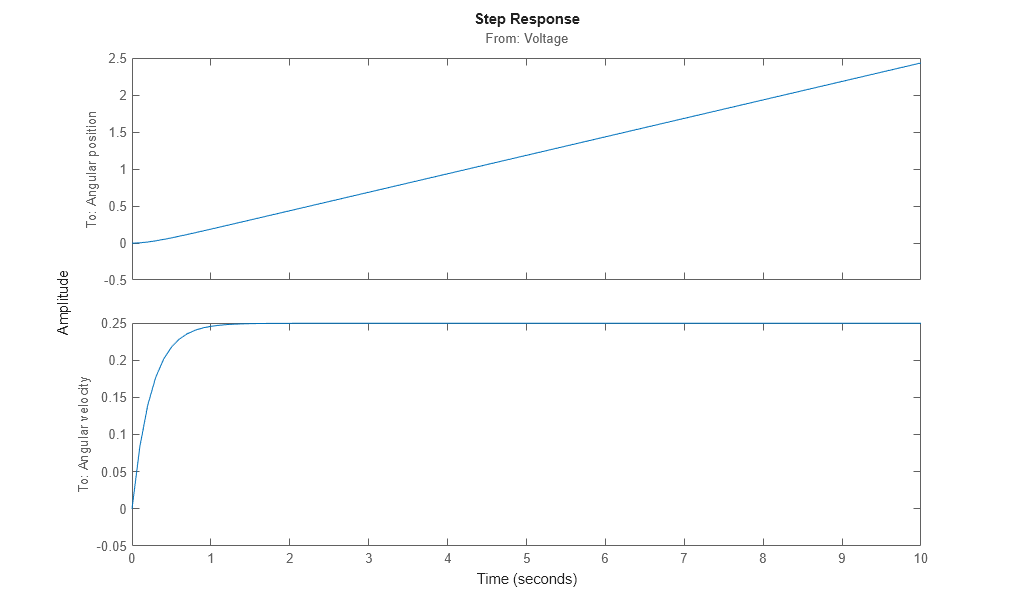Figure 8: Step response with the estimated IDNLGREY DC-motor model.

7. Examine the model covariance.

You can assess the quality of the estimated model to some extent by looking at the estimated covariance matrix and the estimated noise variance. A "small" value of the `(i, i)` diagonal element of the covariance matrix indicates that the `i`:th model parameter is important for explaining the system dynamics when using the chosen model structure. Small noise variance (covariance for multi-output systems) elements are also a good indication that the model captures the estimation data in a good way.

```getcov(nlgr) nlgr.NoiseVariance ```
```ans = 1.0e-04 * 0.1573 0.0021 0.0021 0.0008 ans = 0.0010 -0.0000 -0.0000 0.0110 ```

For more information about the estimated model, use `present` to display the initial states and estimated parameter values, and estimated uncertainty (standard deviation) for the parameters.

```present(nlgr); ```
``` nlgr = Continuous-time nonlinear grey-box model defined by 'dcmotor_m' (MATLAB file): dx/dt = F(t, u(t), x(t), p1, p2) y(t) = H(t, u(t), x(t), p1, p2) + e(t) with 1 input(s), 2 state(s), 2 output(s), and 2 free parameter(s) (out of 2). Inputs: u(1) Voltage(t) [V] States: Initial value x(1) Angular position(t) [rad] xinit@exp1 0.0302675 (estimated) in [-Inf, Inf] x(2) Angular velocity(t) [rad/s] xinit@exp1 -0.133777 (estimated) in [-Inf, Inf] Outputs: y(1) Angular position(t) [rad] y(2) Angular velocity(t) [rad/s] Parameters: Value Standard Deviation p1 Time-constant [s] 0.243649 0.00396671 (estimated) in [-Inf, Inf] p2 Static gain [rad/(V*s)] 0.249644 0.000284486 (estimated) in [-Inf, Inf] Name: DC-motor Status: Termination condition: Change in cost was less than the specified tolerance.. Number of iterations: 5, Number of function evaluations: 6 Estimated using Solver: ode45; Search: lsqnonlin on time domain data "DC-motor". Fit to estimation data: [98.34;84.47]% FPE: 0.001096, MSE: 0.1187 More information in model's "Report" property. <a href="matlab:if exist('nlgr','var'), if isa(nlgr,'idnlgrey'), disp(' '); disp(get(nlgr)), else disp(' '); disp('Unable to display properties for variable nlgr because it is no longer a/an idnlgrey object.');, end, else matlab.graphics.internal.getForDisplay('nlgr'), end">Model Properties</a> ```

### Conclusions

This example illustrates the basic tools for performing nonlinear grey-box modeling. See the other nonlinear grey-box examples to learn about:

• Using nonlinear grey-box models in more advanced modeling situations, such as building nonlinear continuous- and discrete-time, time-series and static models.

• Writing and using C MEX model-files.

• Handling nonscalar parameters.

• Impact of certain algorithm choices.

For more information on identification of dynamic systems with System Identification Toolbox, visit the System Identification Toolbox product information page.